# ML 101: Computer Vision (10 pts)

## What You Need

• A Web browser

## Purpose

To practice making simple machine learning code in Python.

In a browser, go to

From the menu, click File, "New notebook".

## Recognizing Clothing Items

We'll use the Fashion-MNIST dataset of images of clothing. Each example is a 28x28 grayscale image, associated with a label from 10 classes, such as "Coat", "Shirt", and "Bag".

The image below shows some of the images.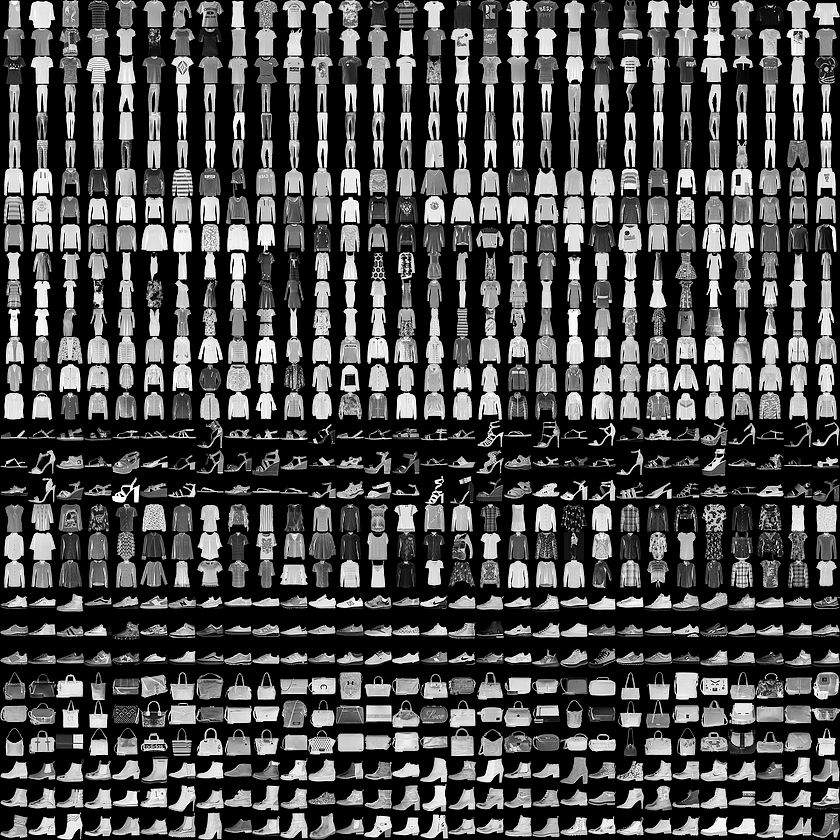Here are the ten classes: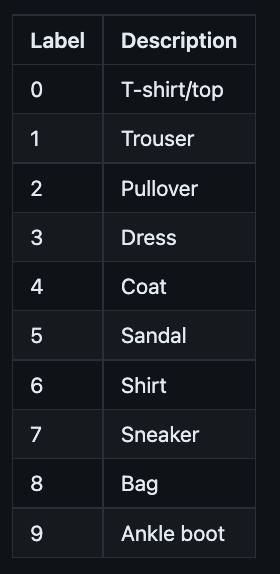Each image is a set of 784 values (28 × 28) between 0 and 255. These act as X values.

To solve this problem, we need a more complex neural network, as shown below. (Image from the textbook in the "Sources" at the bottom of this page.)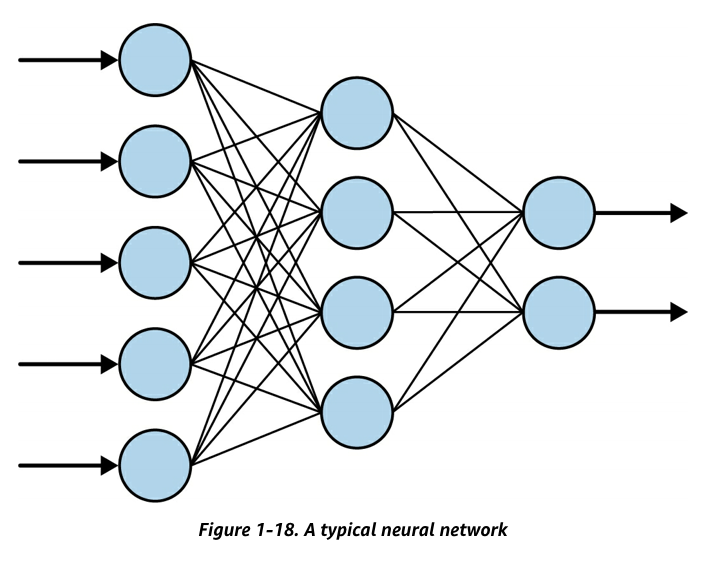## Planning a Neural Network to Recognize Fashion Items

We'll make a neural network with these features, as shown below (in a simplified diagram):
• The inputs are images, consisting of a grid of 28x28 pixels
• The first layer contains 128 neurons (called a "hidden" layer)
• The second layer contains 10 neurons (called the "output" layer)
The output neuron with the strongest signal is the measured category of the image.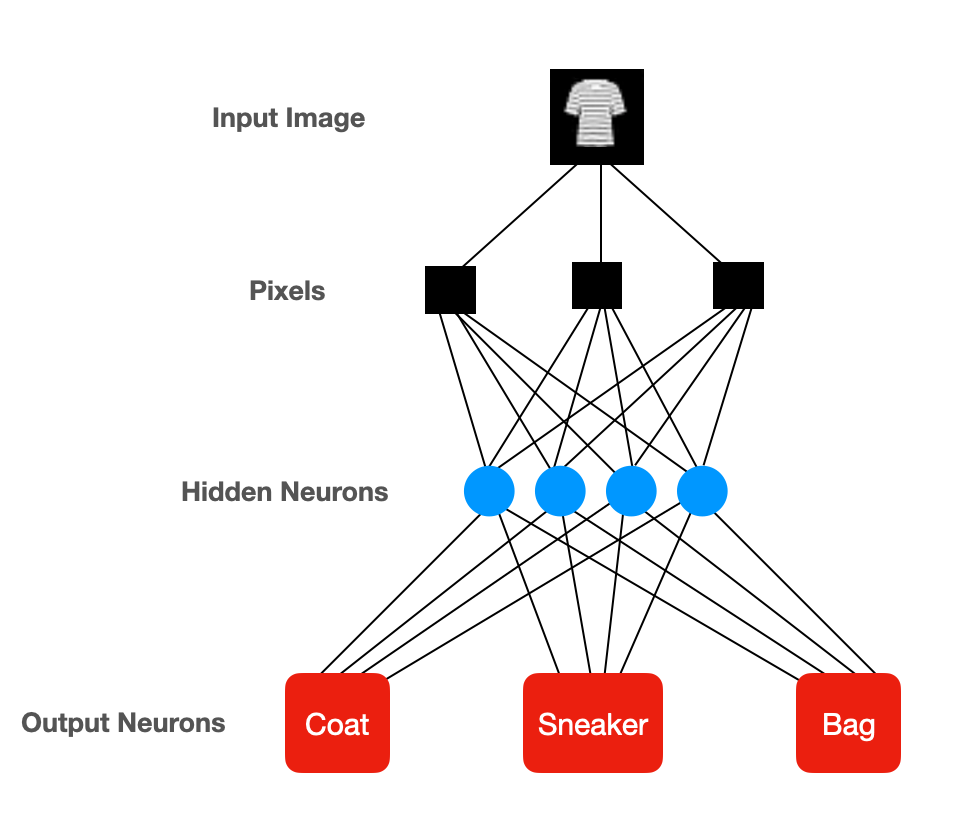## Training the Neural Network

Enter the code below:
```import tensorflow as tf data = tf.keras.datasets.fashion_mnist (training_images, training_labels), (test_images, test_labels) = data.load_data() training_images = training_images / 255.0 test_images = test_images / 255.0 model = tf.keras.models.Sequential([ tf.keras.layers.Flatten(input_shape=(28, 28)), tf.keras.layers.Dense(128, activation=tf.nn.relu), tf.keras.layers.Dense(10, activation=tf.nn.softmax) ]) model.compile(optimizer='adam', loss='sparse_categorical_crossentropy', metrics=['accuracy']) model.fit(training_images, training_labels, epochs=5) model.evaluate(test_images, test_labels) ```
To start training the neural network, click the Run button, outlined in red in the image below.

Notice the two "accuracy" numbers at the bottom right. The first one, outlined in blue, is the accuracy of the model when applied to the training set, which is 0.89 (89%). The second number, outlined in yellow, is the accuracy of the model when applied to the test set, which is 0.87 (87%).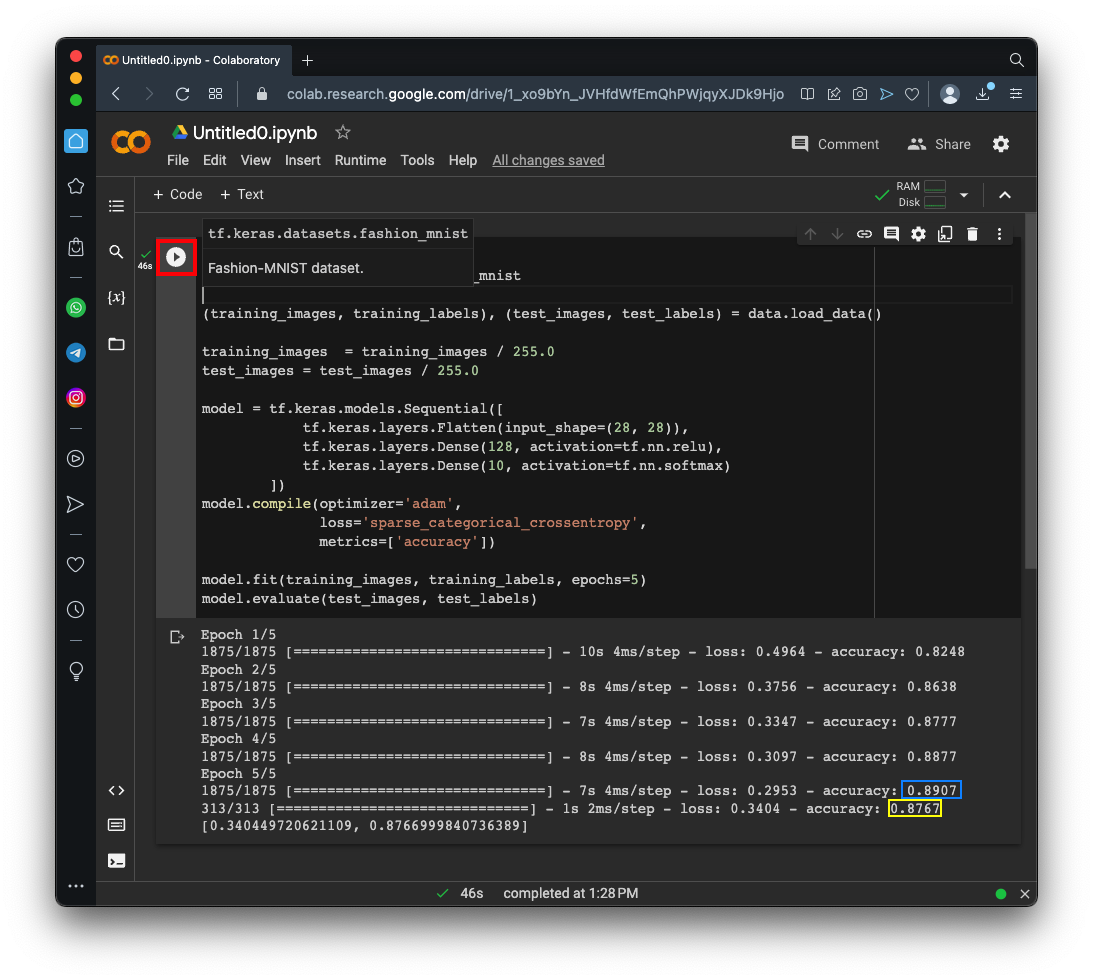## Viewing the Results for 20 Test Images

Delete all the code.

Enter the code below:

```classifications = model.predict(test_images) for i in range(20): print() print(test_labels[i], end = ": ") for c in classifications[i]: print(int(100 * c), end = "\t") ```
Click the Run button.

On each line of the output, the first number is the correct category, and the next ten numbers are the percentages the model puts out for category 0, 1, 2, ... through category 9.

In the image below, look at the first line of the output, outlined in yellow. The correct category is 9, and the network found a 96% probability for column 9. It found the correct category.

Look at the line outlined in red in the image below. Here, the network was confused, giving category 5 a probability of 72% and category 7 a proabability of 27%. The model failed for this image.## Viewing the Images

Delete all the code.

Enter the code below:

```import tensorflow as tf data = tf.keras.datasets.fashion_mnist (training_images, training_labels), (test_images, test_labels) = data.load_data() import matplotlib.pyplot as plt image= test_images fig = plt.figure plt.imshow(image, cmap='gray') plt.show() image= test_images fig = plt.figure plt.imshow(image, cmap='gray') plt.show() ```
Click the Run button.

As you can see, the first image is clearly an Ankle Boot, but the other one could be either a sandal or a sneaker.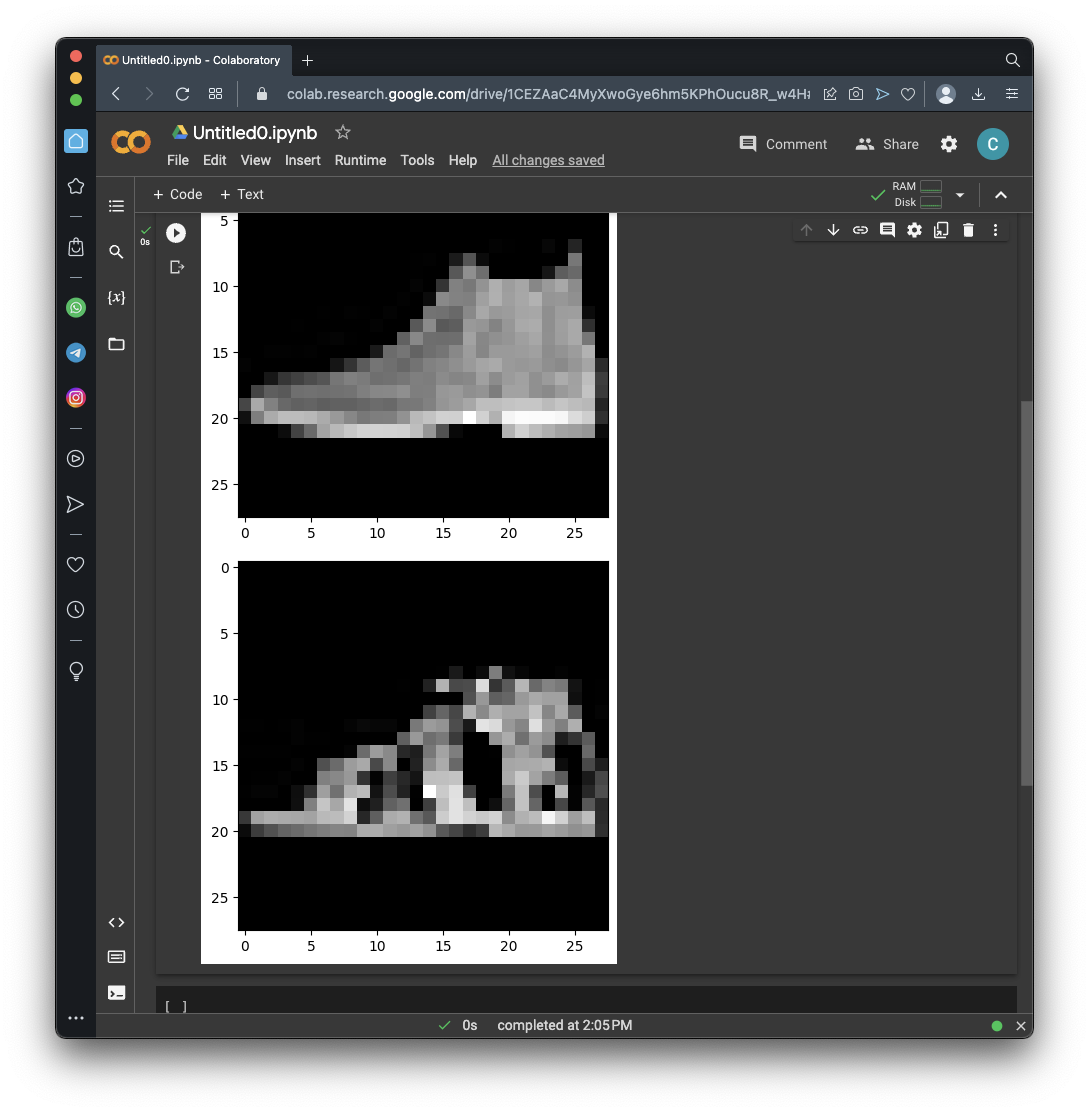## Training for 25 Epochs

Repeat the training, changing the number of epochs in the next-to-last line to 25, as shown below:
```model.fit(training_images, training_labels, epochs=25) ```
As shown below, the accuracy for the training set increased from 89% to 94%, but the accuracy on the test data only increased to 88%. This was only a small improvement.

Would more training improve the model? Perhaps not--sometimes the model becomes too specialized to the training data and actually makes more errors with test data, a problem called overfitting.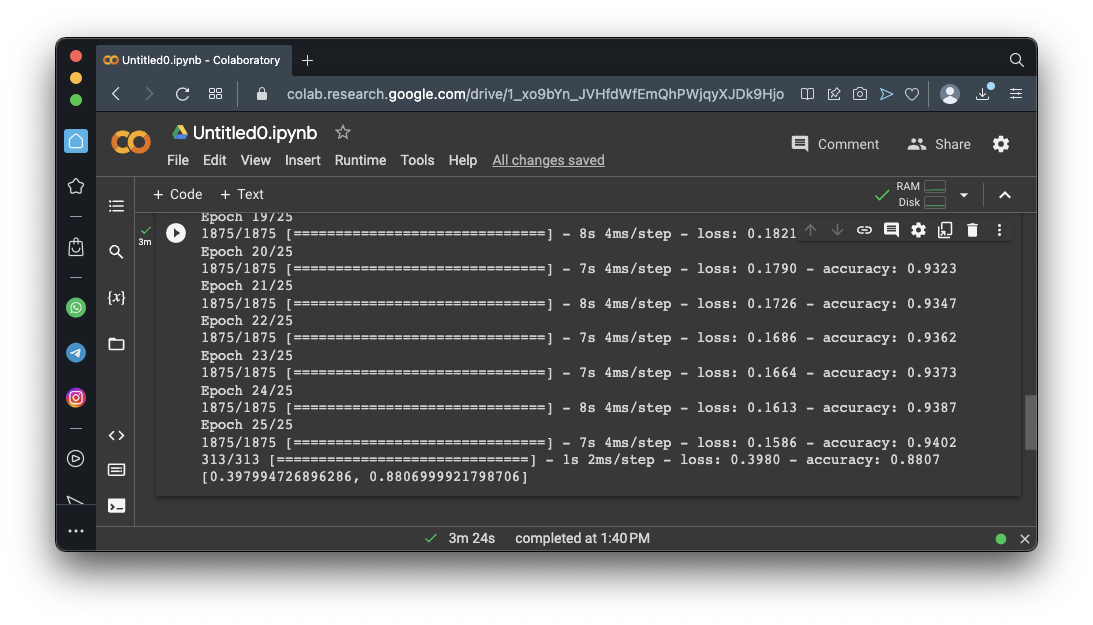## Flag ML 101.1: Learning with Errors (10 pts)

Delete all the code.

Run the code below:

```model.summary() ```
The flag is covered by a green rectangle in the image below.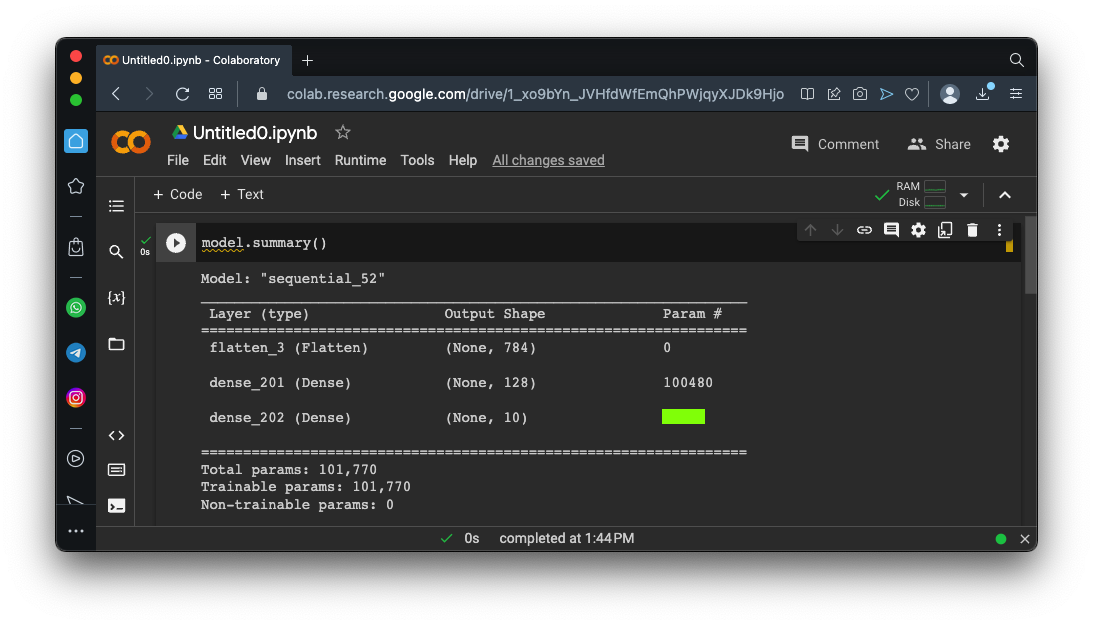## Sources

AI and Machine Learning for Coders: A Programmer's Guide to Artificial Intelligence